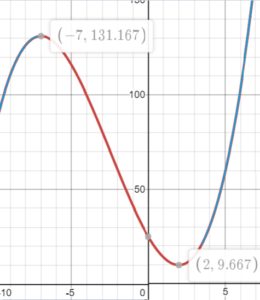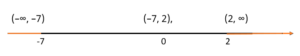# Function Intervals: Decreasing/Increasing

## How do you find function intervals?

You can find the intervals of a function in two ways: with a graph, or with derivatives.

## Find function intervals using a graph

Example Question: Find the increasing intervals for the function g(x) = (⅓)x3 + 2.5x2 – 14x + 25

Step 1: Graph the function (I used the graphing calculator at Desmos.com). This is an easy way to find function intervals. Even if you have to go a step further and “prove” where the intervals are using derivatives, it gives you a way to check your answer.The function appears to be increasing in two intervals: (-∞, -7) and (2, ∞).

The problem with just graphing a function is you don’t know what’s happening off the graph. For example, you could zoom out a thousand times and never be quite sure that the graph isn’t going to do something strange, like a sudden drop. To be 100% sure of your answer, check it with the next few steps.

## Find intervals using derivatives

You can think of a derivative as the slope of a function. If the slope (or derivative) is positive, the function is increasing at that point. If it’s negative, the function is decreasing. So to find intervals of a function that are either decreasing or increasing, take the derivative and plug in a few values.

Example Question: Find the increasing function intervals for g(x) = (⅓)x3 + 2.5x2 – 14x.

Step 1: Find the first derivative. For this particular function, use the power rule:
g′(x) = 3*(⅓)x3-1 + 2* 2.5x2-1 – 14x(1-1) + 99(0)
= x2 + 5x – 14

Step 2: Set the derivative equal to zero and solve (factoring often works):
x2 + 5x – 14 = 0
Factoring, we get:
(x – 2)(x + 7) = 0
Solving for x gives:
x = -7, x = 2.

Step 3: Create intervals on the number line with the x-values from Step 3.For this example, we have 3 intervals: (–∞, –7), (–7, 2), (2, ∞).

Step 4: Pick one point in each interval to “test”. You can choose any number in the interval, but you may want to pick a number like 0, 1, or 10 to make your calculations easier. For example, in the interval (–∞, –7), -10 is a good choice. For (–7, 2), let’s go with 0 and for (2, ∞), 10.

Step 5: Plug the values you chose in Step 5 into the derivative formula (from Step 1) to determine if the derivative has a positive or negative value. Our derivative formula is x2 + 5x – 14, so that gives:

• = -102 + 5(-10) – 14 = 36
• = 02 + 5(0) – 14 = -14
• = 102 + 5(10) – 14 = 136

The first derivative tells us that a function is increasing when the values for the first derivative are positive. Therefore, we can conclude that the increasing function intervals are (-∞, -7) and (2, ∞).

CITE THIS AS:
Stephanie Glen. "Function Intervals: Decreasing/Increasing" From StatisticsHowTo.com: Elementary Statistics for the rest of us! https://www.statisticshowto.com/function-intervals-decreasing-increasing/